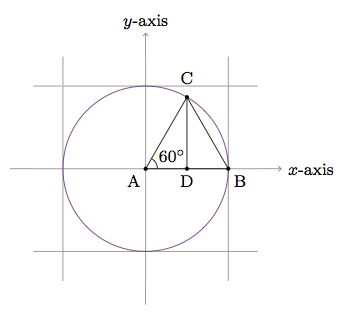# Equilateral triangles and trigonometric functions

Alignments to Content Standards: F-TF.A.3

In the picture below, the purple circle is the set of points in the plane whose distance from the origin (marked as $A$) is 1, often called the unit circle:Also pictured are some other points: $B$ lies on the $x$-axis and the unit circle, $C$ lies on the unit circle, and $D$ is the midpoint of $\overline{AB}$. Angle $\angle BAC=60^\circ$.

1. Show that $\triangle ABC$ is equilateral and conclude that $\overleftrightarrow{CD}$ is perpendicular to $\overleftrightarrow{AB}$.
2. Use part (a) to find the values of $\sin{60^\circ}$ and $\cos{60^\circ}$.
3. Consider the following picture where $Q$ and $R$ are on the unit circle and $S$ is the intersection point of $\overleftrightarrow{QR}$ and the $x$-axis:Show that $\triangle PQR$ is equilateral and that $S$ is the midpoint of $\overline{QR}$.

4. Use part (c) to find the values of $\sin{30^\circ}$ and $\cos{30^\circ}$.

## IM Commentary

The purpose of this task is to apply knowledge about triangles to calculate the sine and cosine of 30 and 60 degrees. Once the calculations of $\sin{60^\circ}$ and $\cos{60^\circ}$ have been made in part (b), there many ways to approach the calculations in part (d). Two methods are presented in the solution. Another nice approach is to reflect the picture from parts (a) and (b) over the line $y = x$. This interchanges the $x$ and $y$ coordinates. In terms of angles, the ray $\overrightarrow{AC}$, which makes a 60 degree angle with the $x$-axis, reflects to a ray making a 30 degree angle with the $x$-axis, giving us the expected relationship between sine and cosine of complementary angles.

Many variants are possible on the arguments given in the solution. For example, in part (c), reflection over the $x$-axis maps the circle to itself and interchanges the two 30 degree angles given in the picture. This means that reflection about the $x$-axis interchanges $Q$ and $R$, making the $x$-axis the perpendicular bisector of $\overline{QR}$. Another argument would show that $\triangle QPS$ and $\triangle RPS$ are congruent (via SAS for example). In part (a) also there are many different arguments for showing that $\overleftrightarrow{CD}$ is perpendicular to $\overleftrightarrow{AB}$.

## Solution

1. Sides $\overline{AB}$ and $\overline{AC}$ both have unit length and this means that $\triangle ABC$ is isosceles with congruent angles $\angle B$ and $\angle C$. Since $m(\angle A)$ is given as 60 this means that $m(\angle B) + m(\angle C) = 120$ and so $m(\angle B) = m(\angle C) = 60$. Hence $\triangle ABC$ is equilateral. Equilateral triangles have three lines of reflective symmetry, the lines joining each vertex to the midpoint of the opposite side. This means that $\overleftrightarrow{CD}$ is a line of symmetry for $\triangle ABC$ and so $\overleftrightarrow{CD}$ is perpendicular to $\overline{AB}$.

2. We apply the Pythagorean Theorem to the right triangle $ADC$, giving us $$|AD|^2 + |CD|^2 = |AC|^2.$$ We saw in part (a) that $D$ is the midpoint of $\overline{AB}$. Since $B$ is on the unit circle, $|AB| = 1$ and $|AD| = \frac{1}{2}$. We have $|AC| = 1$ since $C$ is on the unit circle. Plugging the values for $|AD|$ and $|AC|$ into the displayed formula gives $|CD| = \frac{\sqrt{3}}{2}$. Since $\overleftrightarrow{CD}$ is perpendicular to $\overline{AB}$ we have $$C = \left(\frac{1}{2},\frac{\sqrt{3}}{2}\right).$$ This means that $\sin{60^\circ} = \frac{\sqrt{3}}{2}$ and $\cos{60^\circ} = \frac{1}{2}$.

3. As in part (a), since sides $\overline{PQ}$ and $\overline{PR}$ both have unit length, this means that $\triangle PQR$ is isosceles with congruent angles $\angle Q$ and $\angle R$. Since $m(\angle P) = 60$ it follows that $m(\angle Q) = m(\angle R) = 60$ and $\triangle PQR$ is equilateral.

In an equilateral triangle, each angle bisector is the perpendicular bisector of the opposite side. We are given that $\overrightarrow{PS}$ bisects angle $P$. Hence $S$ is the midpoint of $\overline{QR}$ and $\overleftrightarrow{PS}$ is perpendicular to $\overline{QR}$.

4. As in part (b) above, since $\overleftrightarrow{PS}$ is perpendicular to $\overline{QR}$, the coordinates of $Q$ are $\left(|PS|,|QS|\right)$. We know that $|QS| = \frac{1}{2}$ because $S$ is the midpoint of $\overline{QR}$ and all sides of the triangle $PQR$ have length 1 unit. Applying the Pythagorean theorem to right triangle $PSQ$ we find that $|QS| = \frac{\sqrt{3}}{2}$. Thus $\sin{30^\circ} = \frac{1}{2}$ and $\cos{30^\circ} = \frac{\sqrt{3}}{2}$.

It is also possible to find $\sin{30^\circ}$ and $\cos{30^\circ}$ directly from the calculations in part (b), using the right triangle definition of trigonometric ratios:

\begin{align} \sin{30^\circ} &= \sin{\angle ACD} \\ &= \frac{|AD|}{|AC|} \\ &= \cos{\angle A} \\ &= \frac{1}{2}. \end{align}

So $\sin{30^\circ} = \frac{1}{2}$. We find similarly that $\cos{30^\circ} = \frac{\sqrt{3}}{2}$:

\begin{align} \cos{30^\circ} &= \cos{\angle ACD} \\ &= \frac{|CD|}{|AC|} \\ &= \sin{\angle A} \\ &= \frac{\sqrt{3}}{2}. \end{align}

In each case, rather than using the corresponding trigonometric ratio for the 60 degree angle, we could also use the side lengths that have already been calculated.